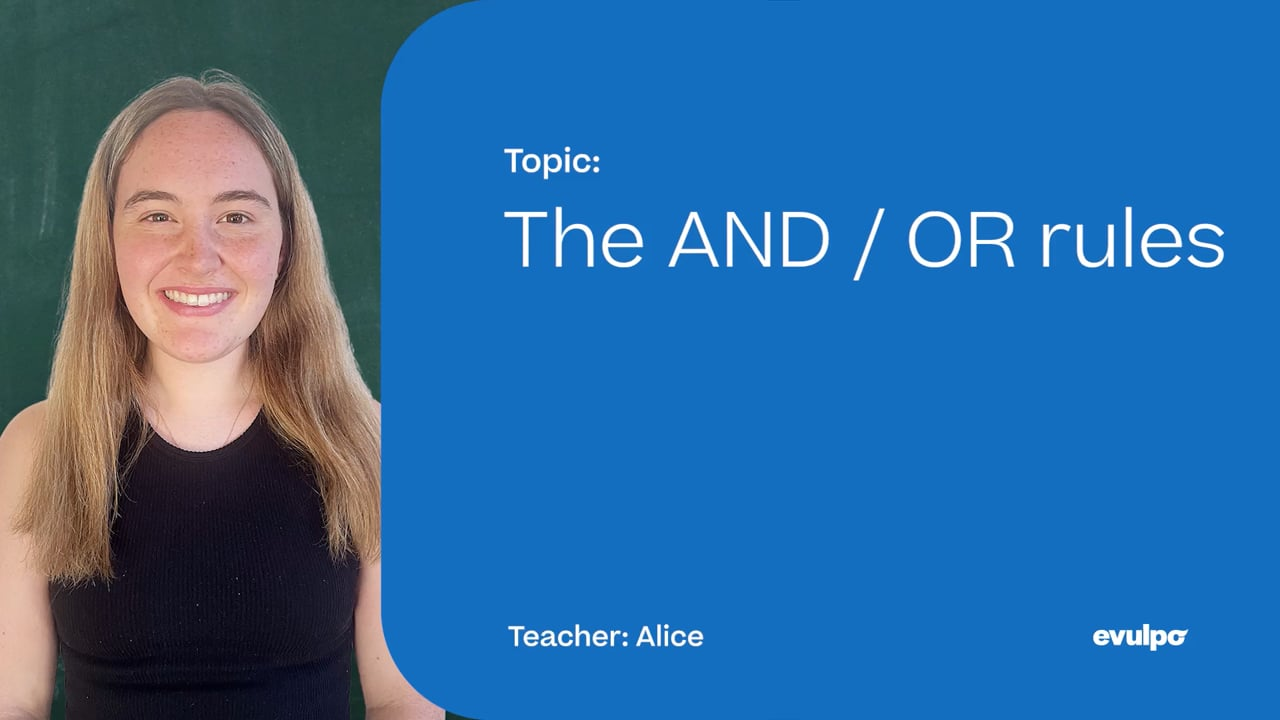Chapter overviewMaths

Exam board

AQA

Number

Algebra

Graphs

Ratio proportion and rates of change

Shapes and area

Angles and geometry

Trigonometry

Probability

Statistics

Maths

# The AND / OR rules0%

Summary

# The AND / OR rules

## In a nutshell

Events can be combined using either of the phrases "and" or "or". For two events A and B, the combined event A AND B occurs if both A happens and B happens. The combined event A OR B happens if either A happens or B happens (at least one must occur).

## AND events

### Definition

Two events are independent if the outcome of either event doesn't affect the other.  If the outcome of one event is affected by the outcome of another, then the events are dependent.

If two events A and B are independent then the AND rule can be used to calculate the probability of the combined event A AND B:

$P(A \text{ and } B)=P(A) \times P(B)$

This formula cannot be used if there A and B are not independent.

##### Example 1

What is the probability of landing heads on a fair coin flip and rolling a 6 on a fair die?

$P(\text{Landing heads}) = \dfrac{1}{2}$

$P(\text{Rolling 6}) = \dfrac{1}{6}$

As these events are independent:

$P(\text{Landing heads and rolling }6)=P(\text{Landing heads})\times P(\text{Rolling }6)=\dfrac{1}{2} \times \dfrac{1}{6} = \underline{\dfrac{1}{12}}$

## OR events

### Definition

Two events are mutually exclusive if the events cannot both occur at the same time. This means that for two mutually exclusive events A and B, $P(A\text{ and }B) = 0$ as there is zero chance of the outcomes both occurring.

For any two events A and B, which are not mutually exclusive, the OR rule can be used to calculate the probability of the combined event A OR B:

$P(A\text{ or }B) = P(A) +P(B) - P(A\text{ and }B)$

For the case where A and B are mutually exclusive we obtain the OR rule as:

$P(A\text{ or }B) = P(A) + P(B)$

##### Example 2

What is the probability of landing a heads on a fair coin flip or rolling a 6 on a fair die?

Rolling a die and flipping a coin are not mutually exclusive events.  Therefore

$P(\text{Landing heads and rolling }6) = \dfrac{1}{12}$

Hence, using the OR rule, $P(\text{Landing heads or rolling }6) = P(\text{Landing heads})+P(\text{Rolling }6)-P(\text{Landing heads and rolling }6) = \dfrac{1}{2} + \dfrac{1}{6} - \dfrac{1}{12} = \underline{\dfrac{7}{12}}$

FAQs

• Question: Can independent events happen at the same time?

Answer: Independent events are unrelated events. The outcome of one event does not impact the outcome of the other event. Independent events can, and do often, occur together.

• Question: How do you know if two probabilities are mutually exclusive?

Answer: Two events are mutually exclusive if they cannot occur at the same time, and so the probability of both events occurring is 0.

• Question: Are disjoint and mutually exclusive events the same thing?

Answer: Two events are mutually exclusive if they cannot occur at the same time. Another word that means mutually exclusive is disjoint. If two events are disjoint, then the probability of them both occurring at the same time is 0.

Theory

Exercises